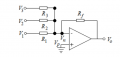# Summing amplifier

#### electronicstudent0371

Joined Nov 24, 2021
2
Good evening all,

Could some please point me in the right direction for the question below: -

"For the summing amplifier, obtain values of R1, R2, R3 and Rf that achieve the following output Vo. Assume that the minimum input resistance is 30 kΩ. Show the formulas used and all working out. = −[51 + 22 + 3]"

Am correct in thinking Rf is either 150Kohms or 60KOhms? I can then use Vo = -(rf/r1*V1 + rf/r2*V2 + Rf/r3*V3) to calculate for r1, r2, r3.Any help would be greatly appreciated, kind regards

#### crutschow

Joined Mar 14, 2008
31,151
What Vo is needed for given values of V1, V2, and V3?

#### electronicstudent0371

Joined Nov 24, 2021
2
What Vo is needed for given values of V1, V2, and V3?
Crutschow, Not been given any values just the equation V0 = - (5V1 + 2V2 + V3)

#### Papabravo

Joined Feb 24, 2006
19,617
Crutschow, Not been given any values just the equation V0 = - (5V1 + 2V2 + V3)
Does that imply that Vo = 7.6 Volts DC? You had to make me work for that one. Did you leave out the decimal points in your 1st post on purpose, or was that an....accident?

#### crutschow

Joined Mar 14, 2008
31,151
Crutschow, Not been given any values just the equation V0 = - (5V1 + 2V2 + V3)
Okay. I didn't see that info in your first post.

So your equation for Vo in the first post is correct.
Then what value of Rf is needed so that the minimum input resistance is 30kΩ for those three gains?

#### MrAl

Joined Jun 17, 2014
9,639
Good evening all,

Could some please point me in the right direction for the question below: -

"For the summing amplifier, obtain values of R1, R2, R3 and Rf that achieve the following output Vo. Assume that the minimum input resistance is 30 kΩ. Show the formulas used and all working out. = −[51 + 22 + 3]"

Am correct in thinking Rf is either 150Kohms or 60KOhms? I can then use Vo = -(rf/r1*V1 + rf/r2*V2 + Rf/r3*V3) to calculate for r1, r2, r3.

View attachment 253360
Any help would be greatly appreciated, kind regards
Hello,

How are you calculating input resistance?
I ask because you are quoting a feedback resistor value that supposedly correlates to the input resistance but the feedback resistor does not influence the input resistance seen by either V1, V2, or V3 without specifying the input voltage (output voltage is already specified).

Your formula is right but i think you need to specify the input voltages too.
If we look at one contribution:
Rf*V1/R1=K1
where we know what K1 is and it is a constant, then we can solve for Rf:
Rf=K1*R1/V1
and if we know that R1 has to be a min of 30 (working in kOhms) then:
Rf=K1*30/V1
or:
Rf=K1*K2/V1
and lumping K1 and K2 into one constant K:
Rf=K/V1
and that's as far as we can go.
Note in some cases i would deem that to be the answer, but in this case the output voltages are given explicitly so i would think we would have to come up with a numerical value for Rf.

That's if i understand your question correctly so far, but if you can elaborate a little that would help here too.

Questions are very very often under specified. I can give a really good example in physics but i might start a new thread for that because it is quite interesting.

#### jlm1948

Joined May 19, 2014
16
This equation " V0 = - (5V1 + 2V2 + V3) " shows that it's an inverting amplifier, where the feedback resistor is 5 times the input resistor for V1. So let's assume R1 is 30k, then Rf is 150k, R2 is 1/2 Rf or 75k and R3 is equal to Rf.
No need to know the voltages; it's algebra.

•RPLaJeunesse

#### MrAl

Joined Jun 17, 2014
9,639
This equation " V0 = - (5V1 + 2V2 + V3) " shows that it's an inverting amplifier, where the feedback resistor is 5 times the input resistor for V1. So let's assume R1 is 30k, then Rf is 150k, R2 is 1/2 Rf or 75k and R3 is equal to Rf.
No need to know the voltages; it's algebra.
Yes if you input 1v to all three inputs, but what if you dont?

#### crutschow

Joined Mar 14, 2008
31,151
Yes if you input 1v to all three inputs, but what if you dont?
What's the difference?
It's a gain equation where the voltages are all relative.

•RPLaJeunesse

#### MrAl

Joined Jun 17, 2014
9,639
What's the difference?
It's a gain equation where the voltages are all relative.
I understand completely what you are saying, but usually when specific values are quoted it means something more specific is being asked so i thought i would query for more information. It's ok with me if everyone wants to take it to work with a gain rather than a specific value i have no problem with that.

#### crutschow

Joined Mar 14, 2008
31,151
usually when specific values are quoted it means something more specific
But there are no specific voltage values quoted, only the gain equation in post #3.

#### MrAl

Joined Jun 17, 2014
9,639
But there are no specific voltage values quoted, only the gain equation in post #3.
Apparently something was changed, look in post #1.